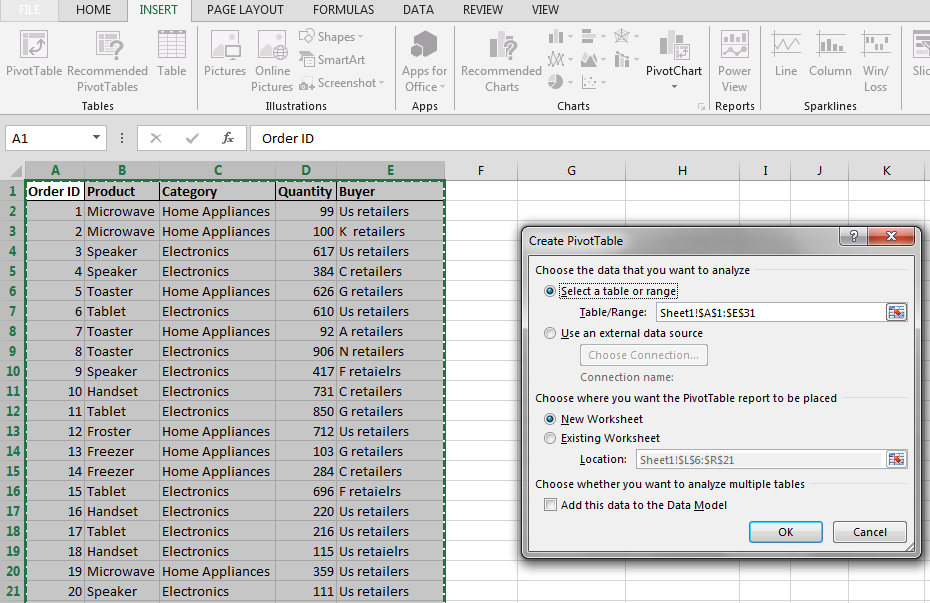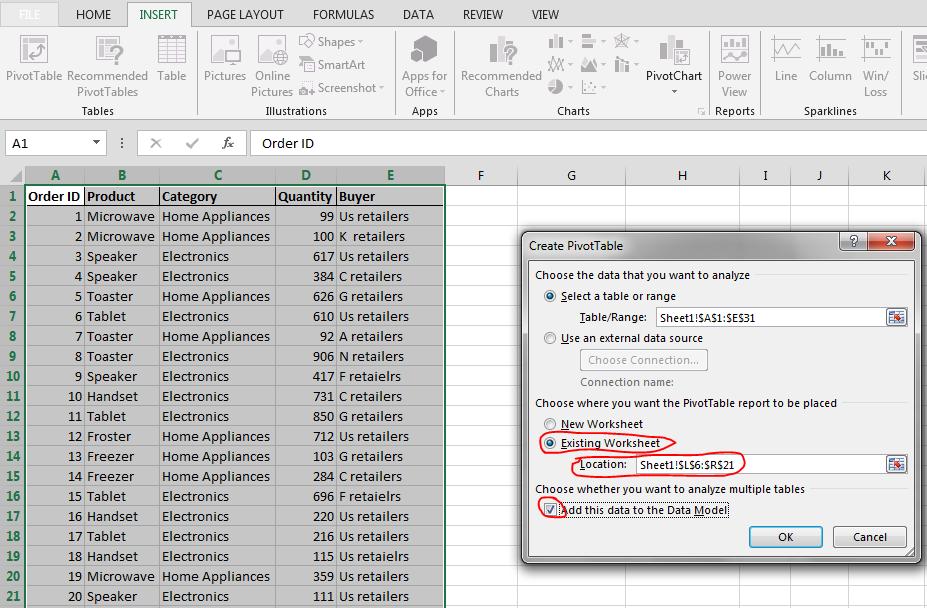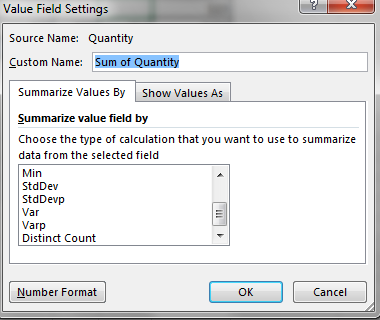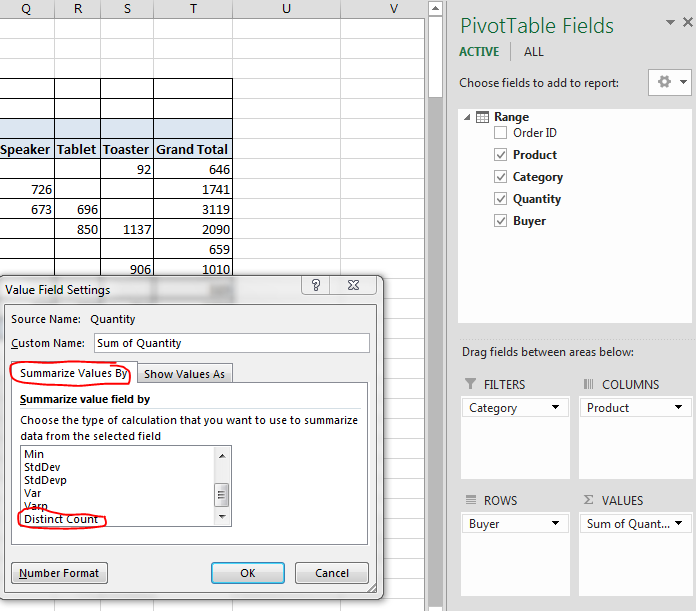#### Counting unique values in pivot table

In this lesson, you will teach yourself how to do counting only distinct values in pivot table. Do you think it is difficult? You will be suprised.

## How to add unique values in pivot table?

In Microsoft Excel, you can count the number of unique values in a pivot table using the following steps:

Once the data is entered into Excel sheet, select the data then go to Insert -> Pivot Table.Select Existing sheet and enter the Location where you want to create the pivot table. Then check the add this value to the Data Model option.## How to count unique values in pivot table?

In the Pivot table Fields pane, drag the fields to lower boxes which are filter, column, rows, and sum of values as per your requirement. Then click the drop down list from Values, select Value Field Settings.In this dialogue, select the tab Summarize Values By and scroll down. Then select Distinct Count. You will see a pivot table which will count only distinct values.## Using a Calculated Field with the COUNTIF Function

To count unique values in a pivot table using a calculated field with the COUNTIF function, follow these steps:

In the PivotTable Fields pane, click the Add Calculated Field button.

In the Create Calculated Field dialog box, enter a name for the field and then type the following formula into the Formula box

`=COUNTIF(field_name, field_name)`

Where field_name is the name of the field that you want to count.

The calculated field will now be added to the PivotTable Fields pane.

Drag the calculated field to the Values area of the pivot table.

The pivot table will now display the number of unique values in the calculated field.

## Which Method Should I Use?

The Distinct Count feature is the easiest way to count unique values in a pivot table. It is also the most efficient method for large datasets.

However, if you need more flexibility in how you count unique values, you can use a calculated field with the COUNTIF function.

For example, you can use a calculated field to count unique values in a subset of the data or to count unique values based on certain criteria.